AP State Syllabus AP Board 7th Class Maths Solutions Chapter 13 Area and Perimeter Ex 6 Textbook Questions and Answers.

## AP State Syllabus 7th Class Maths Solutions 13th Lesson Area and Perimeter Exercise 6Question 1.
A path 2.5 m wide is running around a square field whose side is 45 m. Determine the area of the path.
Solution: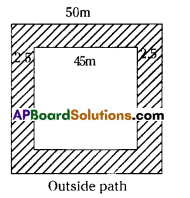Area of the path= (Area of outer figure) – (Area of inner figure)
= (50 × 50) – (45 × 45)
= 2500 – 2025 = 475 m2

Question 2.
The central hail ofa school is 18m long and 12.5 m wide. A carpet is to be laid on the floor leaving a strip 50 cm wide near the walls, uncovered. Find the area of the carpet and also the uncovered portion?
Solution: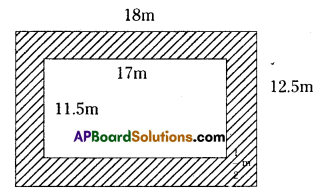Given
Length of the hail = 18m
Width of the strip = 50cm = $$\frac { 1 }{ 2 }$$m
Length of inner rectangle = 18 – ($$\frac { 1 }{ 2 }$$ + $$\frac { 1 }{ 2 }$$) 17m
Breadth of inner rectangle = 12.5 – ($$\frac { 1 }{ 2 }$$ + $$\frac { 1 }{ 2 }$$) = 11.5 m
Area of the strip = (Area of the outer fIgure) – (Area of the inner figure)
= 18 × 12.5 – 17 ×11.5
= 225 – 195.5 = 29.5 m2
∴ Area of the carpet = 195.5 m2Question 3.
The length of the side of a grassy square plot is 80 m. Two walking paths each 4m wide
are constructed parallel to the sides of the plot such that they cut each other at the centre
of the plot. Determine the area of the paths.
Solution: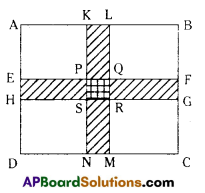Given : A square grassary plot of length 80m
Two paths of width 4m each.
From the question
KL = 4m and KN = 80m
EH = 4m and EF 80m
PQ = 4m and PS 4m
Area of two paths = 🖾 KLMN + 🖾 EFGH – 🖾 FQRS
= KN x KL + EF × EH – PQ × PS
= 80 × 4 + 80 × 4 – 4 × 4
= 320 + 320 – 16
= 624sq.m.Question 4.
A verandah 2 m wide is constructed all around a room of dimensions 8 m X 5 m. Find the area of the verandah
Solution: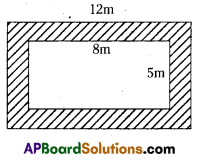Length of outer rectangle = 8 + 2 + 2 =12m
Breadth of outer rectangle = 5 + 2 + 2 = 9m
Area of outer rectangle = l x b = 12 × 9 = 108m2
Length of inner rectangle = 8m
Breadth of inner rectangle = 5m
Area of inner rectangle = l x b = 8 × 5 = 40m2
:. Area of the verandah = (Area of outer figure) – (Area of inner figure)
= 108 – 40 = 68m2Question 5.
The length of a rectangular park is 700 m and its breadth is 300 m. Two crossroads, each of width 10 m, cut the centre of a rectangular park and are parallel to its sides. Find the area of the roads. Also, find the area of the park excluding the area of the crossroads.
Solution: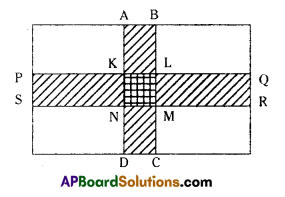Given
Length of the rectangular park = 700 m
Breadth of the rectangular park = 300 ni
From the question,
PS 10m. PQ = 700 ni
Area of 🖾 PQRS = 10 × 700 = 7000m2
AB = 10m, AD = 300m
Area of 🖾 ABCD = 300 x 10 3000m2
KL = 10m, KN = 10m
🖾 KLMN = 10 × 10 = 100m2
Area of the two paths = 🖾 IQRS + 🖾 AF3CD – 🖾 KLMN
= 7000 + 3000 – 100
= 9900m2
The remaining area of the park = Area of the whole park – Area of the two paths
= 700 × 300 – 9900
= 210000 – 9900
= 200100 q.m.